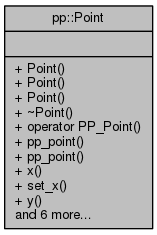Pepper_31_C++_interfaces
pp::Point Class Reference

A 2 dimensional point with 0,0 being the upper-left starting coordinate. More...

`#include <point.h>`

Collaboration diagram for pp::Point:## Public Member Functions

Point ()
The default constructor for a point at 0,0. More...

Point (int32_t in_x, int32_t in_y)

Point (const PP_Point &point)

~Point ()
Destructor. More...

operator PP_Point () const

const PP_Point & pp_point () const

PP_Point & pp_point ()

int32_t x () const

void set_x (int32_t in_x)

int32_t y () const

void set_y (int32_t in_y)

Point operator+ (const Point &other) const

Point operator- (const Point &other) const

Pointoperator+= (const Point &other)

Pointoperator-= (const Point &other)

void swap (Point &other)

## Detailed Description

A 2 dimensional point with 0,0 being the upper-left starting coordinate.

Definition at line 16 of file point.h.

## Constructor & Destructor Documentation

 pp::Point::Point ( )
inline

The default constructor for a point at 0,0.

Definition at line 19 of file point.h.

Referenced by operator+(), and operator-().

 pp::Point::Point ( int32_t in_x, int32_t in_y )
inline

A constructor accepting two int32_t values for x and y and converting them to a Point.

Parameters
 [in] in_x An int32_t value representing a horizontal coordinate of a point, starting with 0 as the left-most coordinate. [in] in_y An int32_t value representing a vertical coordinate of a point, starting with 0 as the top-most coordinate.

Definition at line 31 of file point.h.

 pp::Point::Point ( const PP_Point & point )
inline

A constructor accepting a pointer to a PP_Point and converting the PP_Point to a Point. This is an implicit conversion constructor.

Parameters
 [in] point A pointer to a PP_Point.

Definition at line 40 of file point.h.

 pp::Point::~Point ( )
inline

Destructor.

Definition at line 46 of file point.h.

## Member Function Documentation

 pp::Point::operator PP_Point ( ) const
inline

A function allowing implicit conversion of a Point to a PP_Point.

Returns
A Point.

Definition at line 51 of file point.h.

 Point pp::Point::operator+ ( const Point & other ) const
inline

Adds two Points (this and other) together by adding their x values and y values.

Parameters
 [in] other A Point.
Returns
A new Point containing the result.

Definition at line 99 of file point.h.

References Point(), x(), and y().

 Point& pp::Point::operator+= ( const Point & other )
inline

Adds two Points (this and other) together by adding their x and y values. Returns this point as the result.

Parameters
 [in] other A Point.
Returns
This Point containing the result.

Definition at line 119 of file point.h.

References x(), and y().

 Point pp::Point::operator- ( const Point & other ) const
inline

Subtracts one Point from another Point by subtracting their x values and y values. Returns a new point with the result.

Parameters
 [in] other A Point.
Returns
A new Point containing the result.

Definition at line 109 of file point.h.

References Point(), x(), and y().

 Point& pp::Point::operator-= ( const Point & other )
inline

Subtracts one Point from another Point by subtracting their x values and y values. Returns this point as the result.

Parameters
 [in] other A Point.
Returns
This Point containing the result.

Definition at line 131 of file point.h.

References x(), and y().

 const PP_Point& pp::Point::pp_point ( ) const
inline

Getter function for returning the internal PP_Point struct.

Returns
A const reference to the internal PP_Point struct.

Definition at line 58 of file point.h.

 PP_Point& pp::Point::pp_point ( )
inline

Getter function for returning the internal PP_Point struct.

Returns
A mutable reference to the PP_Point struct.

Definition at line 65 of file point.h.

 void pp::Point::set_x ( int32_t in_x )
inline

Setter function for setting the value of x.

Parameters
 [in] in_x A new x value.

Definition at line 77 of file point.h.

 void pp::Point::set_y ( int32_t in_y )
inline

Setter function for setting the value of y.

Parameters
 [in] in_y A new y value.

Definition at line 89 of file point.h.

 void pp::Point::swap ( Point & other )
inline

Swaps the coordinates of two Points.

Parameters
 [in] other A Point.

Definition at line 140 of file point.h.

References x(), and y().

 int32_t pp::Point::x ( ) const
inline

Getter function for returning the value of x.

Returns
The value of x for this Point.

Definition at line 72 of file point.h.

 int32_t pp::Point::y ( ) const
inline

Getter function for returning the value of y.

Returns
The value of y for this Point.

Definition at line 84 of file point.h.

The documentation for this class was generated from the following file: